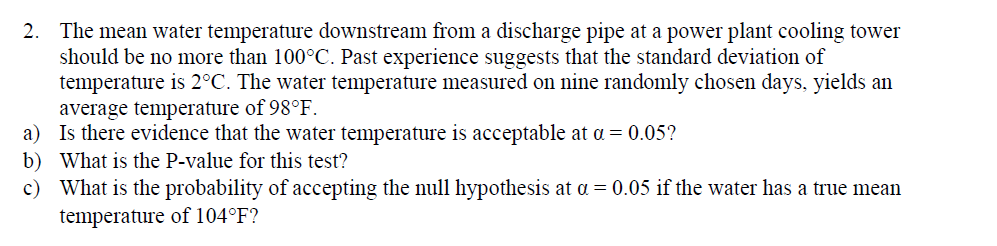# The mean water temperature downstream from a discharge pipe at a power plant cooling towershould be no more than 100°C. Past experience suggests that the standard deviation oftemperature is 2°C. The water temperature measured on nine randomly chosen days, yields anaverage temperature of 98°F2.a) Is there evidence that the water temperature is acceptable at α-0.05?b) What is the P-value for this test?c) What is the probability of accepting the ul hypothesis at a 0.05 if the water has a true meantemperature of 104°F?

Questionhelp_outlineImage TranscriptioncloseThe mean water temperature downstream from a discharge pipe at a power plant cooling tower should be no more than 100°C. Past experience suggests that the standard deviation of temperature is 2°C. The water temperature measured on nine randomly chosen days, yields an average temperature of 98°F 2. a) Is there evidence that the water temperature is acceptable at α-0.05? b) What is the P-value for this test? c) What is the probability of accepting the ul hypothesis at a 0.05 if the water has a true mean temperature of 104°F? fullscreen
check_circle

Step 1

Given that water temperature measured on 9 randomly chosen days is normally distributed with mean 98oF  and standard deviation of temperature is given as 2oF. We need to test if water temperature is acceptable.

Null hypothesis : mean water temperature = 100oF

alternative hypothesis : mean water temperature < 100oF

Here we know population standard deviation so we use a Z test. Test statistic is given by below mentioned formula . y substituting values we get test statistic = -3.

referring to Z table the Z score for probability 0.0505 is -1.64 and Z score for probability 0.4945 is -1.65. So the Z score for probability 0.95 is average of -1.64 and -1.65 = -1.645. At 0.05 significance level the critical Z score is -1.645.

Comparing to test statistic Z score is way less than critical Z score so we reject the null hypothesis.

So we can say at 0.05 significance level the water temperature is less than 100. So the water temperature is acceptable.

Step 2

The p value for Z score -3  is 0.0013 with reference to the Z table shown below.

Step 3

Given that water has true mean temperature of 104oF. we need to find the probability of accepting the null hypothesis.

we accept the nul hypothesis is  p value is greater than 0.05. So the critical score has to be greater than -1.645. The temperature with respect to Z...

### Want to see the full answer?

See Solution

#### Want to see this answer and more?

Solutions are written by subject experts who are available 24/7. Questions are typically answered within 1 hour.*

See Solution
*Response times may vary by subject and question.
Tagged in

### Hypothesis Testing